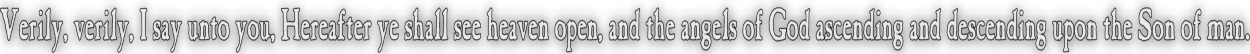None:
Polyps:
Strongs:CreationismBack In The Saddle Again With all this talk of dialectics, I was curious about the first four seals of revelation - as to why the rider was pictured on a "horse" and not as a flying creature or a serpent wriggling out. I considered God's use of word pictures and thought immediately that the one thing a rider and a horse have in common is a saddle. I considered a saddle point, as well as a saddle surface, where the derivative of a function is increasing in one direction and decreasing in another. I thought there may be some topology of the K4 group that related to this geometry. Then I drew a saddle as many maths students have no doubt sketched and saw myself literally use a picture with ∩ and ∪. I thought - this is not at all like a saddle, until it struck me that there are rules for a lattice using meet and join. We have already touched on the Demorgans laws, now I may state that we also have distributive laws. For; A ∩ (B ∪ C) = (A ∩ B) ∪ (A ∩ C) and A ∪ (B ∩ C) = (A ∪ B) ∩ (A ∪ C) Stripping out the elements' symbols in the first case leaves us with (∩ ∪ ∩) And to me at least, this is as simple a "horse" as I can make from these two symbols that also has a "saddle". Also, the distrbutive laws equate this "figure" with " ∩ ( ∪ )" which is "close" to someone saddling a horse viewed from the front of the "horse" with the intersection sign as the saddle, and the union sign as the horses "head". Then it could be said that the horse was saddled so that it "went out" as John stated. We may also mix in Demorgans laws and state comfortably that (A ∩ B)c ∪ (C ∩ D)c = (A ∩ B ∩ C ∩ D)c = (Ac ∪ Bc ∪ Cc ∪ Dc) and (A ∪ B)c ∩ (C ∪ D)c = (A ∪ B ∪ C ∪ D)c = (Ac ∩ Bc ∩ Cc ∩ Dc) Now we know that there is only one rider on all four horses, We could make the identities of A = white, B = red, C = black and D = pale. If we simply send D to the empty set {} then we may choose to have; (A ∩ B)c ∩ (C ∩ D)c = ((A ∩ B) ∪ (C ∩ D))c = ((A ∩ B) ∪ (C ∩ {}))c = (A ∩ B)c And we recover our operation used when sending "wine" to {} Conversely for the oil (D) however, (A ∪ B)c ∪ (C ∪ D)c = (A ∪ B)c ∪Cc = ((A ∪ B) ∩ C)c = ((A ∩C) ∪ (B ∩ C))c Which does not result directly in (A ∪ B)c. Unless of course C is a set that contains both A and B. We should infer that "C" in our case is the synthesis of A and B and in our model of the dialectic system this is the case. So a particular fixed element sent to {}, whether "oil" or "wine" leads to a particular "horse" (∩ ∪ ∩) or its "converse" respectively (when the symbols are "upside down.") ((A ∪ B)c ∪ (C ∪ D)c)c Of course is the synthesis of two syntheses; This results (when D => {}) in; ((A ∪ B)c ∪ (C ∪ D)c)c = (C ∪ Cc)c = {} Now, if this is true in general for four symbols A to D then it is true also no matter which symbols are in view. If we have the two syntheses on exchanging oil and wine of; {d,e,f} => {e,f,g} <= {d,f,g} (oil is "d", wine is "f") and {d,e,f} => {d,e,g} <= {d,f,g} (oil is "f", wine is "d") Then the syntheses of our two syntheses should result in either {d,e,f} or {d,f,g} as in the dialectic "OR" operation from earlier. Yet since one of our operands is sent to {} the synthesis reaching d = {e,f,g} say would be null. Then our "OR" resolves that a synthesis of an inconsistency is again inconsistent. Or rather, either {e,f,g} or {d,e,g} must be "inconsistent". (whilst the pair {d,e,f} and {d,f,g} were supposedly consistent to begin; but one "element" is sent to {}.) Thus one of {d,e,f} and {d,f,g} must also be inconsistent; for (A ∪ B)c if B=>{} implies the complement of A. Then we appear to have an "OR" that is "well trained". "A" would become the excluded middle of C and D; The disjunction of C and D, (the complement of A) is therefore shown "true" by B=>{}. Likewise, ONE of {e,f,g} and {d,e,g} is sent to {}, so for the dialectic "NOT" we would appear to also have a "well trained" operator. When we considered what was meant by "AND" we simply used the synthesis itself. The result is that the choice of element being sent to {} is arbitrary, it is merely as to whether the discourse sides with one position of synthesis or the other - then we may state that (A ∪ B)c = C when D=>{} is "well trained" as an "AND" in that either C or D is null when one is sent to {} and excluded. If C = ¬D in the previous sense then if D =>{} we should state D proves the disjunction of A "XOR" B and C proves the conjunction A "AND" B. Lastly when considering the dialectic "XOR" we could only state that one of C and D must be treated as nullity. Then C "XOR" ¬C whence ¬C = D. Continue To Next Page Return To Section Start Return To Previous Page'